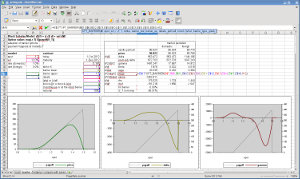## Barrier option pricing within the Black-Scholes model in C++

#### Aims

• analytical barrier pricing functions within the Black-Scholes model
• single and double barriers, continuous barriers/barriers at maturity, barrier and touch options
• analytical calculation of price sensitivities (Greeks)
• C++ in a functional form so it could be quickly ported to C

#### Source Code

The source code is released under the GPLv2 or above:

#### Usage

```// put/call option
price = bs::putcall(S,vol,r,rf,T,K,pc);
delta = bs::putcall(S,vol,r,rf,T,K,pc,bs::types::Delta);
// barrier option, knock in/out, barrier continuously observed or at maturity only
price = bs::barrier(S,vol,r,rf,T,K,B1,B2,rebate,pc,kio,bcont);
vega  = bs::barrier(S,vol,r,rf,T,K,B1,B2,rebate,pc,kio,bcont,bs::types::Vega);

// binary option: cash or nothing (domestic), asset or nothing (foreign)
price = bs::binary(S,vol,r,r,T,B1,B2,fd);
// touch/no-touch option
price = bs::touch(S,vol,r,rf,T,B1,B2,fd,kio,bcont);

// probability that the asset at maturity S_T is in-the-money (irrespective of the path and if it may have hit a barrier before)
prob = bs::prob_in_money(S,vol,mu,T,K,B1,B2,pc);

// probability that the asset S_t hits any of the barriers at any time in [0,T]
prob = bs::prob_hit(S,vol,mu,T,B1,B2);

// Note, if any of the barriers B1 or B2 are set to zero then it is assumed that barrier does not exist.
// Therefore, barrier() can also price vanilla put/call options and touch() can also price cash/asset-or-nothing options.
// putcall(), binary(), bincash(), etc are still provided for convenience.
```

The main barrier pricing functions have been integrated into LibreOffice since version 4.0. They are available as OPT_BARRIER() and OPT_TOUCH().Unfortunately, gnumeric maintainers were not so keen and the code did not get included.

#### Benchmark

The benchmark below shows the number of cpu-cycles used by each pricing function. These numbers are approximate and cpu-architecture- and input parameter dependent. Here it is run on an Intel Core i5-750 Nehalem. The first three functions are only for comparison and the first function gives an idea about the overhead of the benchmark (dummy always returns 0) due to virtual function calls, etc.
```\$ ./bench 2667 1000	# this cpu clocks at 2.667GHz, run 1000k loops

number of cpu-cycles per function:

price  delta  gamma  theta   vega  volga  vanna    rho  rho_f
dummy          10     10     10     10     10     10     10     10     10
add            11     11     11     11     11     11     12     11     11
norm cdf       48     49     48     48     48     48     48     48     48

bincash()     299    355    357    505    355    356    357    395    360
binary()      318    373    374    520    371    370    377    413    375
1 touch()     983   1414   1808   1384   1506   2134   2412   1619   1528
2 touch()   15930  21079  26884  22407  37250  66878  54912  42026  37791

putcall()     393    263    316   1102    837    844    851    895    891
T barrier()  1312   1634   1537   2124   1520   1540   1542   1617   1615
1 barrier()  3542   6202   8027   5797   5890   8041  10173   6105   6301
2 barrier() 27245  37057  44508  46825  71090 128084 105030  76324  76943
```
Source: Compile as follows:
```\$ g++ -Wall -O2 bench.cpp -o bench.o
\$ g++ bench.o bs.o -o bench
```

#### Examples

```#include <cstdio>
#include <cstdlib>
#include "bs.h"

int main(int argc, char** argv) {
// define market data
double S=1.0, vol=0.15, r=0.03, rf=0.01;
// define call option
double T=1.0, K=1.0;
bs::types::PutCall pc = bs::types::Call;
// define barrier option (up-and-out)
double B1=0.0, B2=1.2, rebate=0.0;
bs::types::BarrierKIO kio = bs::types::KnockOut;
bs::types::BarrierActive bcont = bs::types::Continuous;

double price, delta, prob, bhit;

// calculate call option price
price=bs::putcall(S,vol,r,rf,T,K,pc);
delta=bs::putcall(S,vol,r,rf,T,K,pc,bs::types::Delta);
prob=bs::prob_in_money(S,vol,r-rf,T,K,0.0,0.0,pc);
printf("call option: price = %f, delta = %f\n", price, delta);
printf("   probability of S_T in the money    = %.2f%%\n\n", prob*100.0);

// calculate barrier option price
price=bs::barrier(S,vol,r,rf,T,K,B1,B2,rebate,pc,kio,bcont);
delta=bs::barrier(S,vol,r,rf,T,K,B1,B2,rebate,pc,kio,bcont,bs::types::Delta);
prob=bs::prob_in_money(S,vol,r-rf,T,K,B1,B2,pc);
bhit=bs::prob_hit(S,vol,r-rf,T,B1,B2);
printf("barrier option: price = %f, delta = %f\n", price, delta);
printf("   probability of S_T in the money    = %.2f%%\n", prob*100.0);
printf("   probability of hitting the barrier = %.2f%%\n\n", bhit*100.0);

return EXIT_SUCCESS;
}
```
Compile:
```\$ g++ -c -Wall -O2 bs.cpp
\$ g++ -c -Wall -O2 demo.cpp
\$ g++  demo.o bs.o -o demo
\$ ./demo
call option: price = 0.068926, delta = 0.576720
probability of S_T in the money    = 52.33%

barrier option: price = 0.019619, delta = 0.023680
probability of S_T in the money    = 39.97%
probability of hitting the barrier = 24.04%
```
Another example compares the analytical results of the price sensitivities vs finite difference approximation: